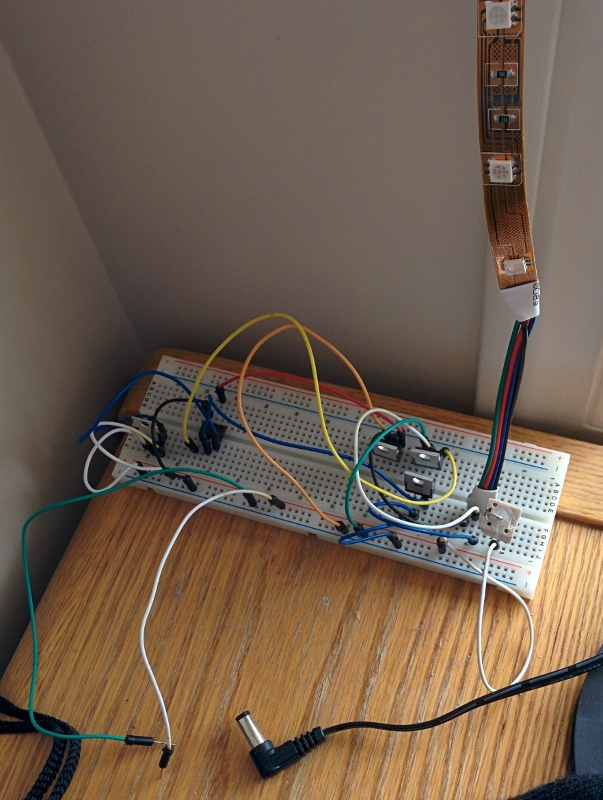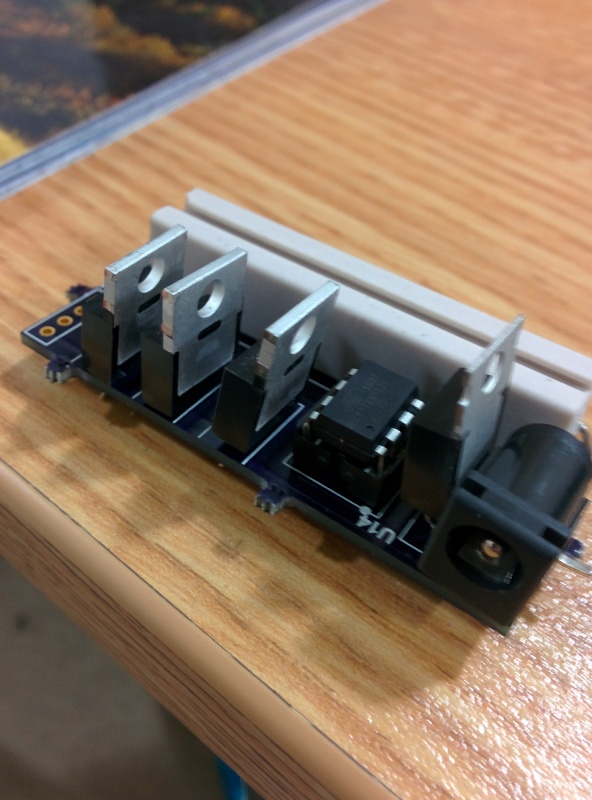This was a quick project to set up a set of high current RGB LED christmas lights. I bought the light strip and needed to create a circuit board to control them.

There are two portions to this project. The first is the schematic and PCB for the light controllers and the second is the code to run on an ATTiny13 micro. It takes 12V in on the barrel plug, and that's about it! It should be able to handle at least a few amperes of current.

### Schematic and PCB

You can see these on Upverter at RGB-Christmas-Lights.

### ATTiny13 Code


#include <avr/io.h>
#include <util/delay.h>

#define LED_RED PB0
#define LED_BLU PB1
#define LED_GRN PB2

void merrychristmas();
void dot(int pin);
void dash(int pin);
void space();
void pwm8000_q(int pin);
void pwm8000_e(int pin);
void pwm8000_s(int pin);

int main(void) {

// Configure LED_PINS as output
DDRB |= (1 << LED_RED);
DDRB |= (1 << LED_BLU);
DDRB |= (1 << LED_GRN);

PORTB |= (1 << LED_RED);
PORTB |= (1 << LED_BLU);
PORTB |= (1 << LED_GRN);

while (1) {
merrychristmas();
//pwm8000_q(LED_BLU);
pwm8000_e(LED_BLU);
pwm8000_s(LED_BLU);
}

return 0;
}

void pwm8000_q(int pin) {

//this will pwm at 1/4 power for 8000 ms
int i=0;
for(i=0; i<250; i++) {
PORTB &= ~(1 << pin);
_delay_ms(4);
PORTB |= (1 << pin);
_delay_ms(12);
}

}
void pwm8000_e(int pin) {

//this will pwm at 1/8 power for 8000 ms
int i=0;
for(i=0; i<250; i++) {
PORTB &= ~(1 << pin);
_delay_ms(2);
PORTB |= (1 << pin);
_delay_ms(14);
}

}

void pwm8000_s(int pin) {

//this will pwm at 1/16 power for 8000 ms
int i=0;
for(i=0; i<250; i++) {
PORTB &= ~(1 << pin);
_delay_ms(1);
PORTB |= (1 << pin);
_delay_ms(15);
}

}

void merrychristmas() {

//spells out merry christmas in morse :)
dash(LED_RED); dash(LED_RED); //m
space();

dot(LED_BLU); //e
space();

dot(LED_GRN); dash(LED_GRN); dot(LED_GRN); //r
space();

dot(LED_RED); dash(LED_RED); dot(LED_RED); //r
space();

dash(LED_BLU); dot(LED_BLU); dash(LED_BLU); dash(LED_BLU); //y

space(); space(); space();

dash(LED_GRN); dot(LED_GRN); dash(LED_GRN); dot(LED_GRN); //c
space();

dot(LED_RED); dot(LED_RED); dot(LED_RED); dot(LED_RED); //h
space();

dot(LED_BLU); dash(LED_BLU); dot(LED_BLU); //r
space();

dot(LED_GRN); dot(LED_GRN); //i
space();

dot(LED_RED); dot(LED_RED); dot(LED_RED); //s
space();

dash(LED_BLU); //t
space();

dash(LED_GRN); dash(LED_GRN); //m
space();

dot(LED_RED); dash(LED_RED); //a
space();

dot(LED_BLU); dot(LED_BLU); dot(LED_BLU); //s

space(); space(); space();
space(); space(); space();
}

//paris standard timings multiplied by 10
void dot(int pin) {

PORTB &= ~(1 << pin);
_delay_ms(600);
PORTB |= (1 << pin);
//and set a space between now and the next character
_delay_ms(600);
}

void dash(int pin) {

PORTB &= ~(1 << pin);
_delay_ms(1200);
PORTB |= (1 << pin);

//and set a space between now and the next character
_delay_ms(600);
}

void space() {
_delay_ms(1200); //compounded with end-of-seq space we get end-of-letter space 180ms
}


### Photos